# Calculation of the area on which each of the four wheels of a vehicle rests

in StemSocial4 months ago (edited)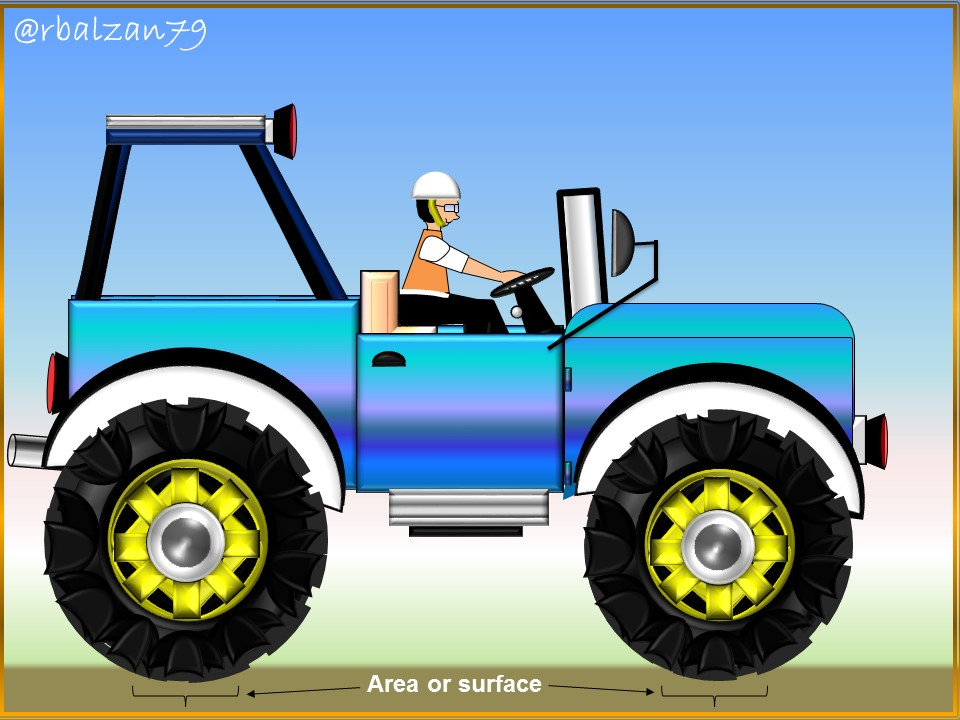Greetings again my dear friends I hope you are all very well, we know that countless are the phenomena that develop around us and, which have influence in our lives and, consequently, in everything we do or do each of the instruments or machines designed by man, one of these phenomena is the pressure in all its dimensions.

Any type of force will exert certain pressure to a certain surface or area, such pressure may be exerted by solid bodies, such as any part of our body or object that surrounds us, by liquid substances (such as water) or by the air that surrounds us, everything can exert pressure to a certain surface or area and, therefore, that is why this time we will relate to a practical exercise that will allow us to link with the phenomenon of pressure, in addition, highlighting with it, which is part of our daily life.

Exercise

Humanity has been able to develop thanks to the creation of valuable machines such as vehicles in any of its presentations, these vehicles have a weight that will exert a pressure on its wheels and, these in turn, will exert a pressure to certain surface or areas where the vehicle is standing on its wheels or tires, therefore, we have a car of 623 kg and whose air pressure in its wheels is 89 kPa, in relation to all that expressed above answer the following question:

a.- What will be the area on which each of the four wheels of our automobile rests?

Solución

Data:

m = 623 Kg (Automobile mass).
Air pressure in the tires = 89 kPa.
g = 9,8 m/s2 (Gravity acceleration).
Wheel areas = ?

a.- To answer our question, we must express that the vehicle will exert a pressure on the ground in relation to the total force of its weight and, for this, we have that: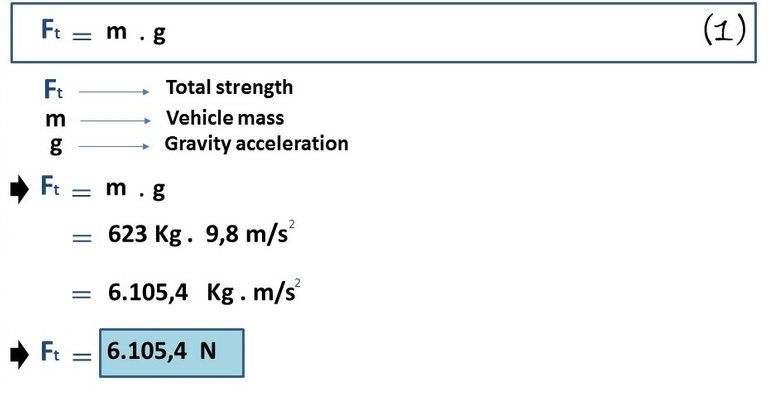In this way we find the value of the total force exerted by the weight of the vehicle, therefore, to know the force on each of the wheels we must divide the total force (Ft), by four which is the number of wheels of our car, so we have: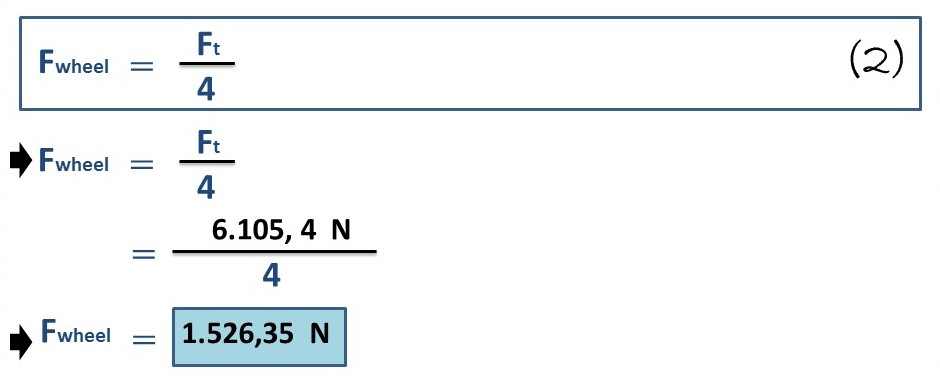We have thus found the particular force exerted by each of the wheels of our analyzed vehicle and, looking at our data, we realize that we have the air pressure of each wheel, then, we can proceed as follows: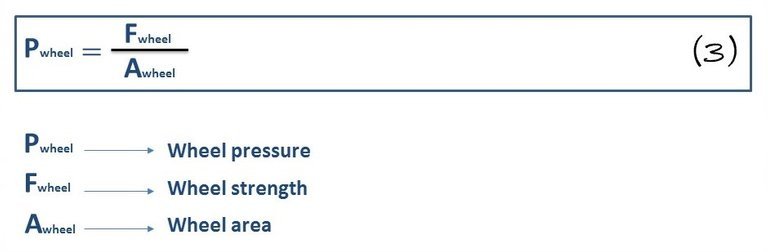Then we proceed to clear the variable of our interest, i.e. Area covered by the wheels, therefore, we are left with: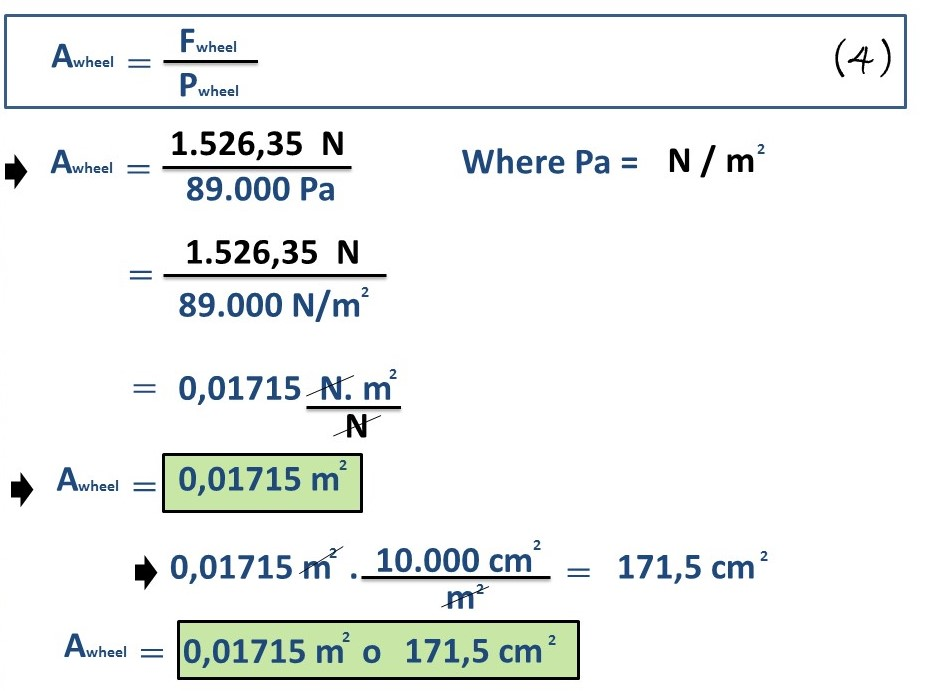In this way, we can obtain the value of the area on which each of the wheels of our analyzed vehicle rests, both in meters and in square centimeters.

# Conclusion

Undoubtedly, we live connected with each of the phenomena that develops around us and, hence, the enormous importance of trying to understand it as best as possible, all this has been possible thanks to the application of science and the implementation of each of the mathematical formulations structured in a general and particular way to these essential phenomena, both of natural and artificial origin.

We live in a world subject to pressure of all kinds, above all, we live in an immense sea of air that exerts pressure on our body and also on the objects that are next to us, in the same way other substances or materials with liquid or solid structures, components of machines such as the vehicle analyzed on this occasion exert pressure on a surface or area which is represented in its four wheels, this is done by its total weight.

Later on, we will continue our search for the application and analysis of other practical everyday examples, where science allows us to explain the development of any type of phenomenon through the implementation of mathematical formulations, as we have done in this opportunity.

Until another opportunity my dear friends.

Note: The image was created by the author using Power Point and Paint.

# Recommended bibliographic references

 PRESSURE AND FLUID STATICS. Link.